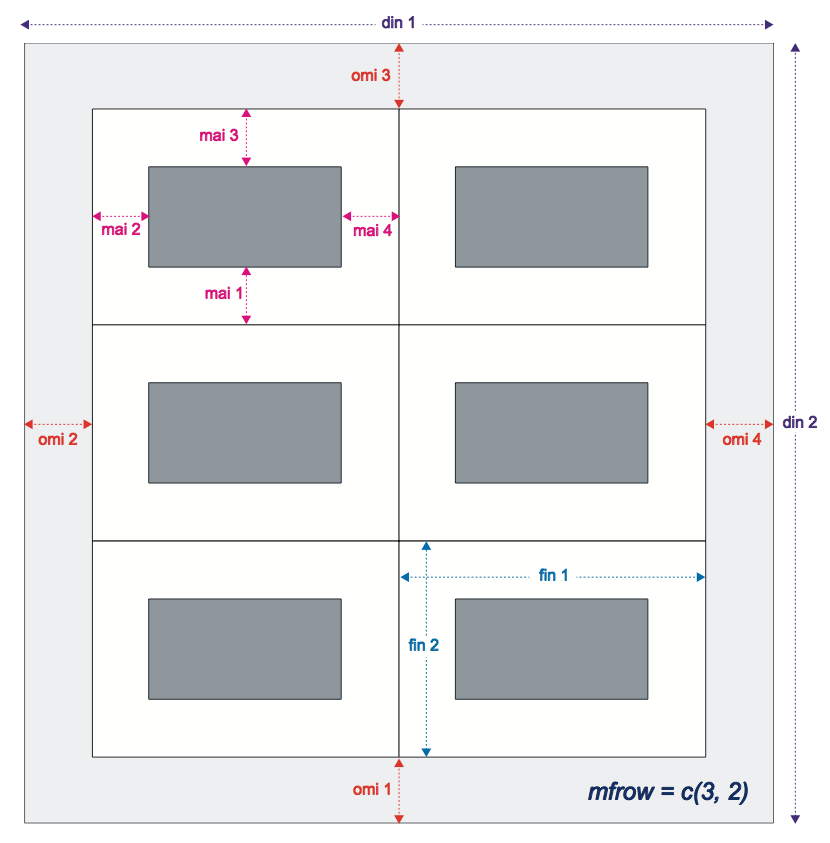# R Graphics: Plot Parameters

High level plot commands open a graphics device.  High level plot elements add objects.  R plot parameters ensure actual control over the graphics device.

#### R Plot Parameters

All high level plotting functions have arguments which can be used to customize the plot. barplot(), for example, has arguments to control bar width, styles, etc.  High level functions also take the optional “three dots” argument, which allows for argument sharing.  The most common arguments shared are low level graph parameters from the par() function.

The tables below define graphical parameters by type.  The most commonly used are highlighted with †.  The example notation c denotes one character for input;  i, j, m, and n are integers; L is a logical value; x requires a numeric data object as input:

#### Layout Parameters

ArgumentDescriptionExample
dinAbsolute device size in inches.c(x1, y1)
figDefine figure region as a fraction of device region.c(x1, x2, y1, y2)
finAbsolute figure size as fraction of the device service.c(width, height)
ftyFigure Type; to control shape of plot; m=(default) maximal; s=square; r=arranged by rows; c=arranged by columns.c
mfg †Multiple figure graphics; gives the row and column count.c(i, j, m, n)
mfcolmfg plots drawn column by column (N-folding ).!ERROR! unexpected comma
mfrowmfg plots drawn row by row (Z-folding).!ERROR! unexpected comma

#### Plot Formatting

ArgumentDescriptionExample
col †Color, device dependent (see notes below for color wheel choices).x
lty †Line Type; 1=solid, 2 and up is dotted or dashed.x
lwd †Line width; 1=standard; all others are multiple of default.x

#### Margins

ArgumentDescriptionExample
maiMargin size in inches.c(xbot, xlef, xtop, xrig)
marMargin size in text font size; default = c(5, 4, 4, 2) + 0.1c(xbot, xlef, xtop, xrig)
mexCo-ordinate unit for addressing locations in the margin.x
omaDefine outer margin in terms of text lines.c(xbot, xlef, xtop, xrig)
omdDefine outer margin as fraction of device region.c(x1,x2,y1,y2)
omiDefine outer margin in terms of inches.c(xbot, xlef, xtop, xrig)

#### Plot Area

ArgumentDescriptionExample
btyType of box; o=full (deflault), l=mar(1,2),7=mar(3,4); c=mar(3,2,1).c
pinWidth and height of plot in inches.c(width, height)
ptyType of plot region; s=square; m=max size (no margins).c
uinInches per usi unit.c(x1, x2)
usrUser coordinate limits (min, max) on the x/y axies: default=c(0,1,0,1).c(x1, x2, y1, y2)
xpdClipping; FALSE means no points/lines outside plot area.L
xlim †Min/max x-axis values.c(x1, x2)
ylim †Min/max v-axis valuesc(y1, y2)

#### Axes

ArgumentDescriptionExample
atPlacement of tick marks; often used with pretty().x
axisIf axes=T, an enclosing box, tick marks and axis labels are plotted.L
expLabels format: 0 is exponential, 1 #s on one line, 2 (default) 2*10^6x
labDesired # of tic intervals on x or y-axis and label lengthc(x, y, llen)
labelsAxis labelsx
lasStyle of axis labels; 0=parallel to axis (default); 1=horiz; 2=perp.x
logMargin for the axis title, labels and line; default=c(3,1,0).c
mgpMargin for the axis title, labels and line; default=c(3,1,0).c(x1, x2, x3)
tck ††Tick mark length; negative=outside the plot region (default=−0.02); if tck=1 then gridlines result; embedded in x or y axis function.x
xaxp / yaxpCo-ordinates lower tick u1, upper tick uh and the # of intervals nc(ul, uh, n)
xaxs / yaxs †Axis interval; s and e are standard and extended (>data values); i is axis label < data values; r range + 4%; d is direct (no change).c
xaxt / yaxtAxis type; n=null; s=standard, t=time, l=log.c

#### Text and Symbols

ArgumentDescriptionExample
cex †Character size and expansion.x
csiFont height.x
expNotation; 0=exp base e, 1=exp one line, 2=exp (default: 2*10^6 format).x
fontFont number (device dependent).i
main †Primary titletitle(main = ”string”)
pch †# of the plot symbol or ‘c’ for character (see format table below).n or c
smoSmoothness of curves; # rasters for straight line aprox; 8 is the min.x
srtString rotation in degrees.x (e.g. 90)
sub †Secondary titletitle(sub = ”string”)
type †type of plot desired (see table above)c
xlab †x-axis labelstring
ylab †y-axis labelstring

#### Anatomy of a Plot

A figure consists of a plot region surrounded by 4 margins:

The fundamental anatomy fields are critical to managing layout parameters.  For example, the following layout diagram outlines parameters for customizing a 3×2 multi-figure graphic where mfg=c(3,2) with outer margin parameters: